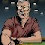## Wednesday, December 26, 2012

### NFL Picks Contest- Results and Standings"Vegas Vic" takes the lead!
Here are the results and updated standings in the NFL Picks Contest

lightning- New England (W 23-16) = 17 pts.
TwoMinuteWarning- Green Bay (W 55-7) = 58 pts.
Grange95-  New England (W 23-16) = 17 pts.
ohcowboy12go- Indianapols (W 20-13) = 17 pts.
Full House- No Pick = 0 pts.
Music City Momma- Indianapolis (W 20-13) = 17 pts.
Coach-  No Pick = 0 pts.
Waffles- No Pick = 0 pts.
MOJO-  New England (W 23-16) = 17 pts.
ggrouchie- New England (W 23-16) = 17 pts.
Vegas Vic-  Green Bay (W 55-7) = 58 pts.
Captain Crunch- Denver (W 34-12) = 32 pts.

Here are the current standings;

Vegas Vic - 16+19+22+13+16+40+15+23+43+58 = 265 pts.
MOJO - 40+19+22+13+16+18+24+68+22+17 = 259
TwoMinuteWarning - 13+19+22+16+31+18+19+23+31+58 = 250
Grange95 - 20+19+22+13+14+40+24+23+43+17 = 235
Music City Momma - 17+19+24+5+17+34+24+24+43+17 =  224
Captain Crunch - 13+11+18+16+31+18+24-(2)+31+32 = 192
lightning - 13+19+24+13+16+18+24+24+22+17 = 190
grrouchie - 14+48+20+5+16+40+17+23-(28)+17 = 172
Coach - 20+19+22+13+16+40+17+38-(28)+0 = 157
ohcowboy12go - 13+19+22+5+13+24+23-(7)+17=129
Waffles - 15+11+22+16+14+0+24-(28)+31+0 =105
Full House - 20+19+22-(34)+16+18+17+24-(7)+0 =95

Congratulations to Vegas Vic for taking the lead!

#### 1 comment:

1.Surprised the grouch hasn't show up to grrouche about GB pickers.